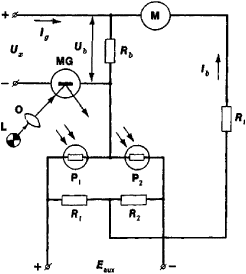# Photoelectric Galvanometer Amplifier, Balanced

The following article is from The Great Soviet Encyclopedia (1979). It might be outdated or ideologically biased.

## Photoelectric Galvanometer Amplifier, Balanced

a DC amplifier used to measure very small currents or voltages. Such amplifiers consist of a magnetoelectric or electrostatic mirror galvanometer, which converts the current or voltage being measured into the deflection of a light beam, and a photoelectric transducer, which converts small deviations (usually fractions of a degree) of the galvanometer mirror into a current or voltage.Figure 1. Schematic of a balanced photoelectric galvanometer amplifier for measuring voltage: (Ux) voltage being measured, (MG) mirror galvanometer, (L) light source, (O) objective lens, (P1) and (P2) photoresistors, (Eaux) auxiliary voltage source, (lg) current in the galvanometer circuit, (lb) current in the load circuit, (R1) and (R2) resistors, (Rb) balance resistor, (R1) load, (Ub) balance voltage, (M) milliammeter

Figure 1 shows a balanced photoelectric galvanometer amplifier for voltage measurements. The voltage Ux generates a current Ig in the galvanometer circuit, and the galvanometer mirror is deflected. The luminous flux is deflected by the mirror onto the photoresistors of the transducer connected in a bridge circuit and is redistributed in such a way that the current in one of the photoresistors increases and the current in the other decreases. As a result, a current difference Ib arises in the load circuit and increases until Ui is compensated (balanced) by a drop in voltage in the balance resistor Ub = Ib · Rb. The value Ux is determined from Ib · Ig and Ib vary correspondingly with a change in Ux (by ∆g and ∆Ib, respectively). The ratio k = ∆Ib/∆Ig is called the coefficient of amplification and ranges from 103 to 108, depending on the design of the instrument.

Instruments of this type may be used to measure voltages from 10–6 to 1 volt and currents from 10–9 to 5 × 10–2 ampere.

### REFERENCES

Rabinovich, S. G. Fotogal’vanometricheskie kompensatsionnye pribory. Moscow-Leningrad, 1964.
Ornatskii, P. P. Avtomaticheskie izmereniia i pribory: (Analogovye i tsifrovye), 3rd ed. Kiev, 1973.

A. V. KOCHEROV